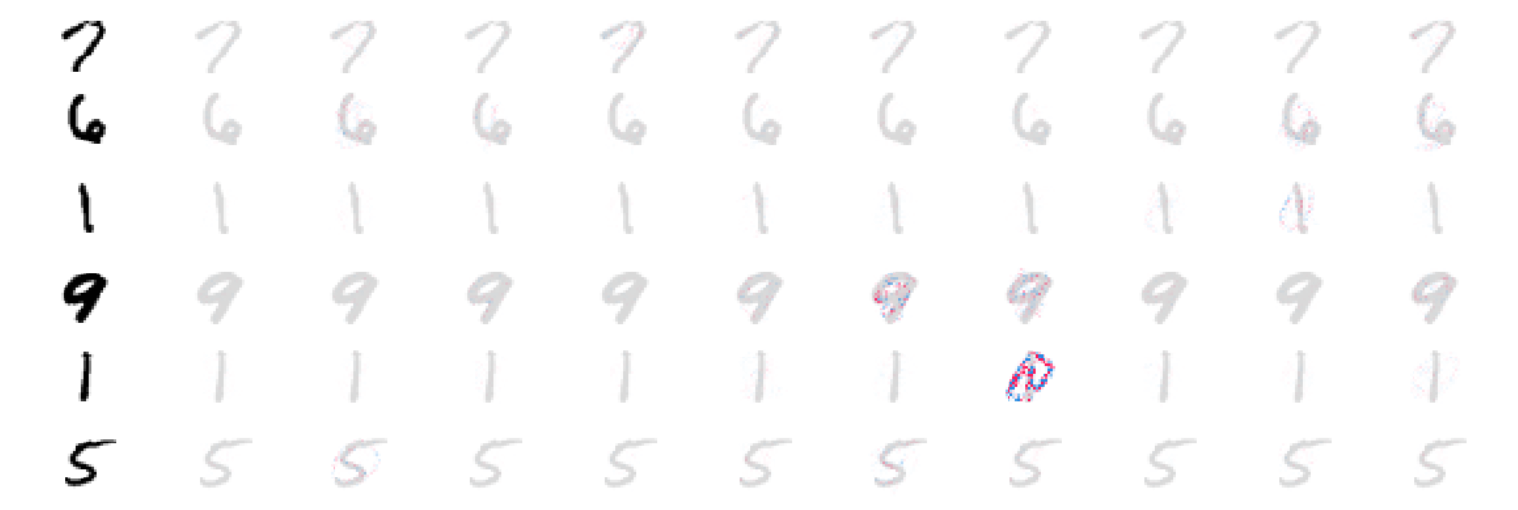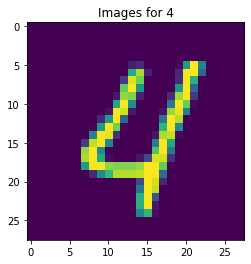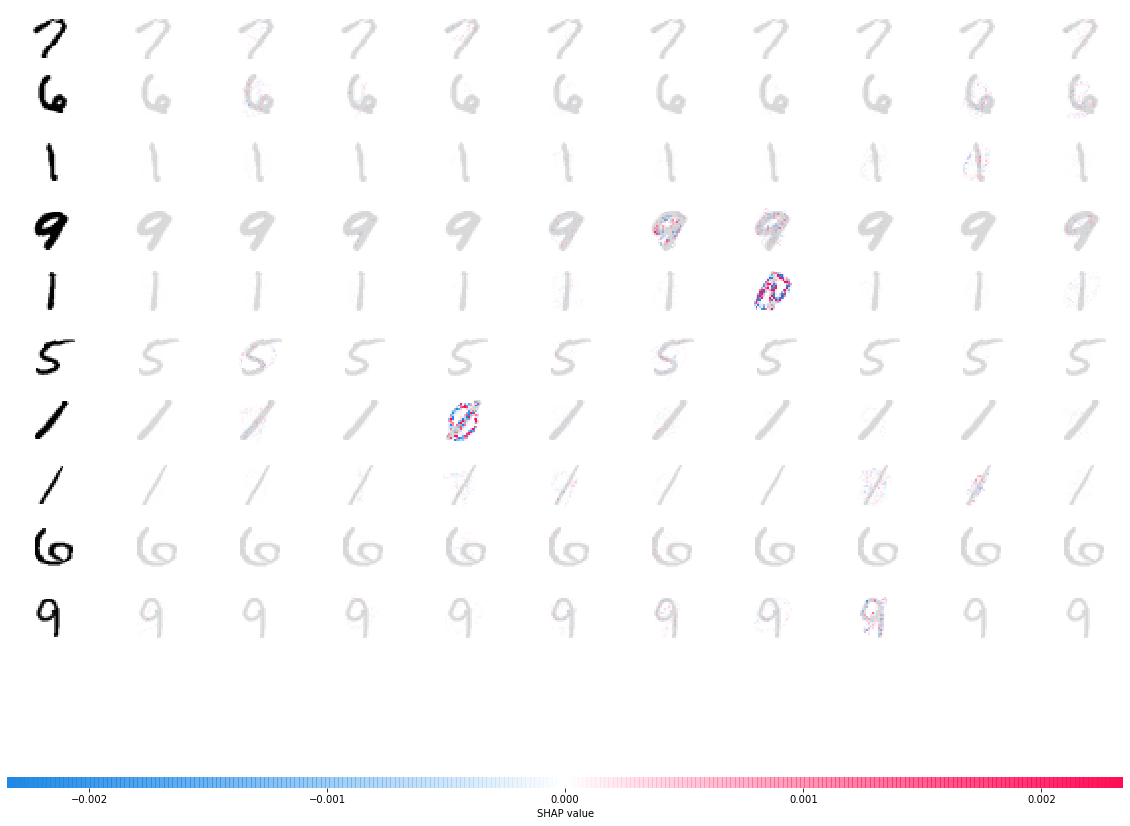# Explain Image Classification by SHAP Deep Explainer

## Goal¶

This post aims to introduce how to explain Image Classification (trained by PyTorch) via SHAP Deep Explainer.

Shap is the module to make the black box model interpretable. For example, image classification tasks can be explained by the scores on each pixel on a predicted image, which indicates how much it contributes to the probability positively or negatively.Reference

## Libraries¶

In :
import torch, torchvision
from torchvision import datasets, transforms
from torch import nn, optim
from torch.nn import functional as F
from torchviz import make_dot
import numpy as np
import shap
import matplotlib.pyplot as plt
%matplotlib inline


## Deep Learning Model Preparation¶

### Configuration¶

In :
batch_size = 128
num_epochs = 2
learning_rate = 0.01
momentum=0.5
device = torch.device('cpu')


### Network Definition¶

In :
class Net(nn.Module):
def __init__(self):
super(Net, self).__init__()

# Convolution Layers
self.conv_layers = nn.Sequential(
nn.Conv2d(1, 10, kernel_size=5),
nn.MaxPool2d(2),
nn.ReLU(),
nn.Conv2d(10, 20, kernel_size=5),
nn.Dropout(),
nn.MaxPool2d(2),
nn.ReLU(),
)

#
self.fc_layers = nn.Sequential(
nn.Linear(320, 50),
nn.ReLU(),
nn.Dropout(),
nn.Linear(50, 10),
nn.Softmax(dim=1)
)

def forward(self, x):
x = self.conv_layers(x)
x = x.view(-1, 320)
x = self.fc_layers(x)
return x

In :
model = Net()
model

Out:
Net(
(conv_layers): Sequential(
(0): Conv2d(1, 10, kernel_size=(5, 5), stride=(1, 1))
(1): MaxPool2d(kernel_size=2, stride=2, padding=0, dilation=1, ceil_mode=False)
(2): ReLU()
(3): Conv2d(10, 20, kernel_size=(5, 5), stride=(1, 1))
(4): Dropout(p=0.5)
(5): MaxPool2d(kernel_size=2, stride=2, padding=0, dilation=1, ceil_mode=False)
(6): ReLU()
)
(fc_layers): Sequential(
(0): Linear(in_features=320, out_features=50, bias=True)
(1): ReLU()
(2): Dropout(p=0.5)
(3): Linear(in_features=50, out_features=10, bias=True)
(4): Softmax()
)
)

### Define Train & Test procedure¶

The followings are the process in train and test:

• optimizer.zero_grad() - reset the gradients before update (only training)
• output = model(data) - predict the value based on the current model
• F.nll_loss(output.log(), target) - compute the loss. Here we use negative log likelihood.
• loss.backward() - calculate gradients based on the loss
• optimizer.step() - execute backward propagation to update the weights
In :
def train(model, device, train_loader, optimizer, epoch):
model.train()
for batch_idx, (data, target) in enumerate(train_loader):
data, target = data.to(device), target.to(device)
output = model(data)
loss = F.nll_loss(output.log(), target)
loss.backward()
optimizer.step()
if batch_idx % 100 == 0:
print('Train Epoch: {} [{}/{} ({:.0f}%)]\tLoss: {:.6f}'.format(
100. * batch_idx / len(train_loader), loss.item()))

model.eval()
test_loss = 0
correct = 0
data, target = data.to(device), target.to(device)
output = model(data)

# sum up batch loss
test_loss += F.nll_loss(output.log(),
target).item()

# get the index of the max log-probability
pred = output.max(1, keepdim=True)
correct += pred.eq(target.view_as(pred)).sum().item()

print(
'\nTest set: Average loss: {:.4f}, Accuracy: {}/{} ({:.0f}%)\n'.format(


In :
train_loader = torch.utils.data.DataLoader(datasets.MNIST(
'mnist_data',
train=True,
transform=transforms.Compose([transforms.ToTensor()])),
batch_size=batch_size,
shuffle=True)

'mnist_data',
train=False,
transform=transforms.Compose([transforms.ToTensor()])),
batch_size=batch_size,
shuffle=True)

In :
batch = next(iter(train_loader))
images, labels = batch
plt.imshow(images[:1].numpy());
plt.title(f'Images for {str(labels[:1].numpy())}');### Execute training and testing¶

In :
# Instantiate the model and optimizer
model = Net().to(device)
optimizer = optim.SGD(model.parameters(), lr=learning_rate, momentum=momentum)

for epoch in range(1, num_epochs + 1):

Train Epoch: 1 [0/60000 (0%)]	Loss: 2.319488
Train Epoch: 1 [12800/60000 (21%)]	Loss: 2.277828
Train Epoch: 1 [25600/60000 (43%)]	Loss: 1.916413
Train Epoch: 1 [38400/60000 (64%)]	Loss: 1.093477
Train Epoch: 1 [51200/60000 (85%)]	Loss: 0.805137

Test set: Average loss: 0.0046, Accuracy: 8997/10000 (90%)

Train Epoch: 2 [0/60000 (0%)]	Loss: 0.729434
Train Epoch: 2 [12800/60000 (21%)]	Loss: 0.693940
Train Epoch: 2 [25600/60000 (43%)]	Loss: 0.644763
Train Epoch: 2 [38400/60000 (64%)]	Loss: 0.553247
Train Epoch: 2 [51200/60000 (85%)]	Loss: 0.443303

Test set: Average loss: 0.0024, Accuracy: 9429/10000 (94%)



## Create Deep Explainer¶

### Load some images for explainer¶

In :
# since shuffle=True, this is a random sample of test data
images, _ = batch
images.size()

Out:
torch.Size([128, 1, 28, 28])

### Instanciate Deep Explainer with background images (100 imagesm)¶

In :
background = images[:100]
e = shap.DeepExplainer(model, background)
e

Out:
<shap.explainers.deep.DeepExplainer at 0x12f621240>

### Explain the test images¶

In :
n_test_images = 10
test_images = images[100:100+n_test_images]
shap_values = e.shap_values(test_images)

In :
# rehspae the shap value array and test image array for visualization
shap_numpy = [np.swapaxes(np.swapaxes(s, 1, -1), 1, 2) for s in shap_values]
test_numpy = np.swapaxes(np.swapaxes(test_images.numpy(), 1, -1), 1, 2)


## Visualize SHAP value for test images¶

The shap value that indicate the score for each class are shown as below. The rows indicate the test images and the columns are the classes from 0 to 9 going left to right.

Note that the obtained score as below does not make sense against the original example notebook. For example, 5th prediction for "1" has a lot of positive value on "6"

In :
# plot the feature attributions
shap.image_plot(shap_numpy, -test_numpy)